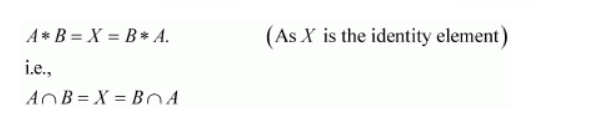# Given a non-empty set X, consider the binary operation *: P(X) × P(X) → P(X) given by A * B = A ∩ B &mnForE;Question:

Given a non-empty set $X$, consider the binary operation *: $P(X) \times P(X) \rightarrow P(X)$ given by $A$ * $B=A \cap B$ \&mnForE; $A, B$ in $P(X)$ is the power set of $X$. Show that $X$ is the identity element for this operation and $X$ is the only invertible element in $\mathrm{P}(X)$ with respect to the operation*.

Solution:

It is given that $*: \mathrm{P}(X) \times \mathrm{P}(X) \rightarrow \mathrm{P}(X)$ is defined as $A^{*} B=A \cap B \forall A, B \in \mathrm{P}(X)$.

We know that $A \cap X=A=X \cap A \forall A \in \mathrm{P}(X)$.

$\Rightarrow A * X=A=X * A \forall A \in \mathrm{P}(X)$

Thus, X is the identity element for the given binary operation *.

Now, an element $A \in \mathrm{P}(X)$ is invertible if there exists $B \in \mathrm{P}(X)$ such thatThis case is possible only when A = X = B.

Thus, X is the only invertible element in P(X) with respect to the given operation*.

Hence, the given result is proved.